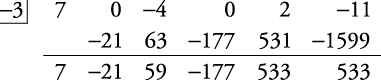## Remainder Theorem

If a polynomial P( x) is divided by ( xr), then the remainder of this division is the same as evaluating P( r), and evaluating P( r) for some polynomial P( x) is the same as finding the remainder of P( x) divided by ( xr).

##### Example 1

Find P(–3) if P( x) = 7 x 5 – 4 x 3 + 2 x –11.

There are two methods of finding P(–3).

Method 1: Directly replace –3 for x.

Method 2: Find the remainder of P( x) divided by [ x – (–3)].

To use Method 1:By Method 2, P( x) divided by [ x – (–3)] will be done by synthetic division.Therefore, P(–3) = –1610.

##### Example 2

Find the remainder of P( x) divided by ( x – 4) if

P( x) = x 4 + x 3 – 13 x 2 – 25 x – 12

By Method 1,By Method 2, synthetic division,Therefore, the remainder = 0.

In Example , since the division has a remainder of zero, both the divisor (the number doing the dividing) and the quotient (the answer) are factors of the dividend (the expression being divided).